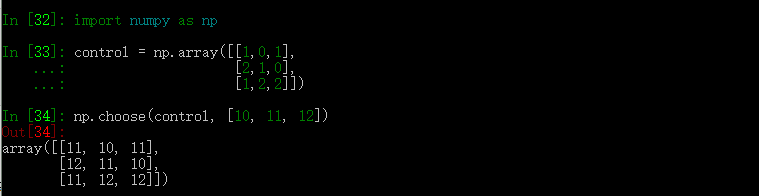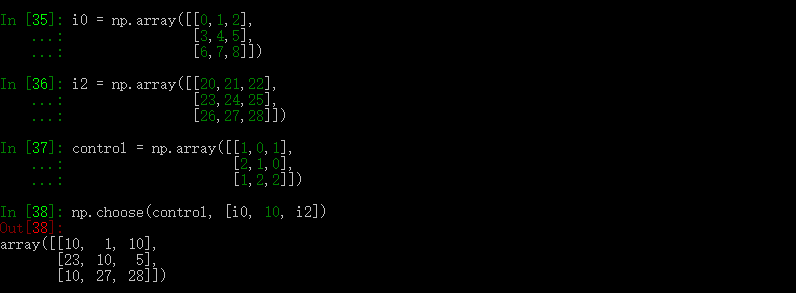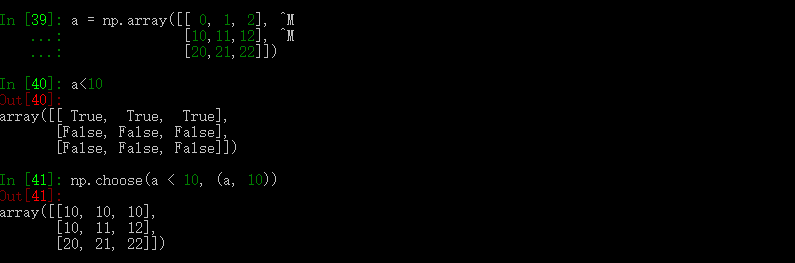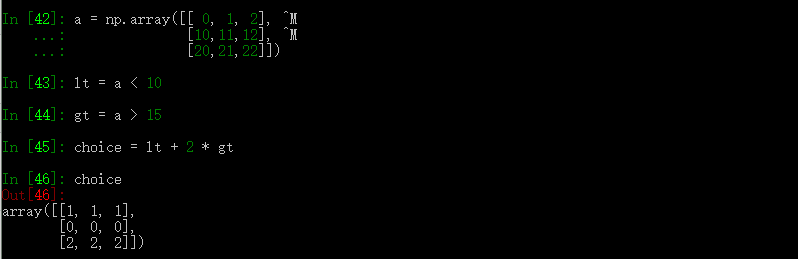• ## EXCEL条件筛选函数

千次阅读 2018-08-21 15:00:56
=IF(ISNUMBER(SEARCH("烟感",B3168)),"001",IF(ISNUMBER(SEARCH("手报",B3168)),"002",IF(ISNUMBER(SEARCH("消火栓",B3168)),"...,IF(ISNUMBER(SEARCH("...
=IF(ISNUMBER(SEARCH("烟感",B3168)),"001",IF(ISNUMBER(SEARCH("手报",B3168)),"002",IF(ISNUMBER(SEARCH("消火栓",B3168)),"003",IF(ISNUMBER(SEARCH("温感",B3168)),"004"))))
展开全文EXCEL IF EXCEL IF
• NumPy 排序、条件刷选函数 NumPy 提供了多种排序的方法。 这些排序函数实现不同的排序算法，每个排序算法的特征在于执行速度，最坏情况性能，所需的工作空间和算法的稳定性。 下表显示了三种排序算法的比较。 ...


NumPy 排序、条件刷选函数
NumPy 提供了多种排序的方法。 这些排序函数实现不同的排序算法，每个排序算法的特征在于执行速度，最坏情况性能，所需的工作空间和算法的稳定性。 下表显示了三种排序算法的比较。

种类速度最坏情况工作空间稳定性'quicksort'（快速排序）
1
O(n^2)
0
否
'mergesort'（归并排序）
2
O(n*log(n))
~n/2
是
'heapsort'（堆排序）
3
O(n*log(n))
0
否

numpy.sort()
numpy.sort() 函数返回输入数组的排序副本。函数格式如下：
numpy.sort(a, axis, kind, order)
参数说明：
a: 要排序的数组
axis: 沿着它排序数组的轴，如果没有数组会被展开，沿着最后的轴排序， axis=0 按列排序，axis=1 按行排序
kind: 默认为'quicksort'（快速排序）
order: 如果数组包含字段，则是要排序的字段

numpy.argsort()
numpy.argsort() 函数返回的是数组值从小到大的索引值。

numpy.lexsort()
numpy.lexsort() 用于对多个序列进行排序。把它想象成对电子表格进行排序，每一列代表一个序列，排序时优先照顾靠后的列。

import numpy as np

nm =  ('raju','anil','ravi','amar')
dv =  ('f.y.',  's.y.',  's.y.',  'f.y.')
ind = np.lexsort((dv,nm))
print ('调用 lexsort() 函数：')
print (ind)
print ('\n')
print ('使用这个索引来获取排序后的数据：')
print ([nm[i]  +  ", "  + dv[i]  for i in ind])

import numpy as np

nm =  ('raju','anil','ravi','amar')

dv =  ('f.y.',  's.y.',  's.y.',  'f.y.')

ind = np.lexsort((dv,nm))

print ('调用 lexsort() 函数：')

print (ind)

print ('\n')

print ('使用这个索引来获取排序后的数据：')

print ([nm[i]  +  ", "  + dv[i]  for i in ind])

调用 lexsort() 函数：
[3 1 0 2]

使用这个索引来获取排序后的数据：
['amar, f.y.', 'anil, s.y.', 'raju, f.y.', 'ravi, s.y.']

上面传入 np.lexsort 的是一个tuple，排序时首先排 nm，顺序为：amar、anil、raju、ravi 。综上排序结果为 [3 1 0 2]。

msort、sort_complex、partition、argpartition

函数描述msort(a)
数组按第一个轴排序，返回排序后的数组副本。np.msort(a) 相等于 np.sort(a, axis=0)。
sort_complex(a)
对复数按照先实部后虚部的顺序进行排序。
partition(a, kth[, axis, kind, order])
指定一个数，对数组进行分区
argpartition(a, kth[, axis, kind, order])
可以通过关键字 kind 指定算法沿着指定轴对数组进行分区

numpy.argmax() 和 numpy.argmin()
numpy.argmax() 和 numpy.argmin()函数分别沿给定轴返回最大和最小元素的索引。

numpy.nonzero()
numpy.nonzero() 函数返回输入数组中非零元素的索引。

numpy.where()
numpy.where() 函数返回输入数组中满足给定条件的元素的索引。

numpy.extract()
numpy.extract() 函数根据某个条件从数组中抽取元素，返回满条件的元素。

import numpy as np

x = np.arange(9.).reshape(3,  3)
print ('我们的数组是：')
print (x)
# 定义条件, 选择偶数元素
condition = np.mod(x,2)  ==  0
print ('按元素的条件值：')
print (condition)
print ('使用条件提取元素：')
print (np.extract(condition, x))

我们的数组是：
[[ 0.  1.  2.]
[ 3.  4.  5.]
[ 6.  7.  8.]]
按元素的条件值：
[[ True False  True]
[False  True False]
[ True False  True]]
使用条件提取元素：
[ 0.  2.  4.  6.  8.]

转载于:https://www.cnblogs.com/DaLinY/p/920a03b7a3df557ec5b83f70985a4b53.html
展开全文• choose 函数实现条件筛选 对于数组，我们有时候需要进行类似 switch 和 case 进行条件选择，此时使用 choose 函数十分方便：   在上面的例子中，choose 将 0,1,2 对应的值映射为了 10, 11, 12，这里的 0,1,2 ...
choose 函数实现条件筛选

对于数组，我们有时候需要进行类似 switch 和 case 进行条件选择，此时使用 choose 函数十分方便：在上面的例子中，choose 将 0,1,2 对应的值映射为了 10, 11, 12，这里的 0,1,2 表示对应的下标。

事实上， choose 不仅仅能接受下标参数，还可以接受下标所在的位置：这里，control 传入第一个 1 对应的是 10，传入的第一个 0 对应于 i0 相应位置的值即 1，剩下的以此类推。

下面的例子将数组中所有小于 10 的值变成了 10。下面的例子将数组中所有小于 10 的值变成了 10，大于 15 的值变成了 15。展开全文• choose 函数实现条件筛选 对于数组，我们有时候需要进行类似 switch 和 case 进行条件选择，此时使用 choose 函数十分方便： import numpy as np control = np.array([[1,0,1], [2,1,0], [1,2,2]]) np.choose...
choose 函数实现条件筛选
对于数组，我们有时候需要进行类似 switch 和 case 进行条件选择，此时使用 choose 函数十分方便：
import numpy as np

control = np.array([[1,0,1],
[2,1,0],
[1,2,2]])

np.choose(control, [10, 11, 12])

array([[11, 10, 11],
[12, 11, 10],
[11, 12, 12]])

在上面的例子中，choose 将 0,1,2 对应的值映射为了 10, 11, 12，这里的 0,1,2 表示对应的下标。
事实上， choose 不仅仅能接受下标参数，还可以接受下标所在的位置：
i0 = np.array([[0,1,2],
[3,4,5],
[6,7,8]])
i2 = np.array([[20,21,22],
[23,24,25],
[26,27,28]])
control = np.array([[1,0,1],
[2,1,0],
[1,2,2]])

np.choose(control, [i0, 10, i2])

array([[10,  1, 10],
[23, 10,  5],
[10, 27, 28]])

这里，control 传入第一个 1 对应的是 10，传入的第一个 0 对应于 i0 相应位置的值即 1，剩下的以此类推。
下面的例子将数组中所有小于 10 的值变成了 10。
a = np.array([[ 0, 1, 2],
[10,11,12],
[20,21,22]])

a < 10

array([[ True,  True,  True],
[False, False, False],
[False, False, False]], dtype=bool)

np.choose(a < 10, (a, 10))

array([[10, 10, 10],
[10, 11, 12],
[20, 21, 22]])

下面的例子将数组中所有小于 10 的值变成了 10，大于 15 的值变成了 15。
a = np.array([[ 0, 1, 2],
[10,11,12],
[20,21,22]])

lt = a < 10
gt = a > 15

choice = lt + 2 * gt
choice

array([[1, 1, 1],
[0, 0, 0],
[2, 2, 2]])

np.choose(choice, (a, 10, 15))

array([[10, 10, 10],
[10, 11, 12],
[15, 15, 15]])



展开全文• filter()函数是 Python 内置的另一个有用的高阶函数，filter()函数接收一个函数 f 和一个list，这个函数 f 的作用是对每个元素进行判断，返回 True或 False，filter()根据判断结果自动过滤掉不符合条件的元素，返回...
• 我的两个DataFrame需要有两个条件进行筛选，此时要用到多条件merge. MultipleKey Merge (基于多个key上的merge) #Dframe on left `python df_left = DataFrame({'key1': ['SF', 'SF', 'LA'], ...python 大数据
• can'kahttps://blog.csdn.net/qq_38727626/article/details/100164430pandas
• ## hive之条件筛选

千次阅读 2019-04-25 10:59:21
常用的条件筛选函数为：if函数，coalesce函数，case when函数 1、if函数（处理单个列的查询结果） 语法：if(condition,value_if_true,value_if_false) 说明：当条件Condition为TRUE时，返回value_True；否则返回...数据库
• Py之pandas：利用isin函数对dataframe格式数据按照多个字段的条件筛选 目录 利用isin函数对dataframe格式数据按照多个字段的条件筛选 代码设计 输出结果 利用isin函数对dataframe格式数据按照...
• 总结一下Filter函数的使用。 Staff表数据如下： 1. 使用多个FILTER实现嵌套筛选
• case when函数基础使用方法 人员编号 1 2019-07-18 00:00:00 1 2019-07-22 00:00:00 1 2019-07-11 00:00:00 2 2019-08-30 00:00:00 2 2019-10-15 00:00:00 2 2019-07-14 00:00:00 3 2019-07-01 00:00:00 3 ...sql case when
• 利用Excel SUBTOTAL函数筛选条件下生成连续序号.rar,本例展示了一份商品进货明细表，利用SUBTOTAL函数可以生成连续1~N的商品序号，同时保证在数据筛选条件下也能够保持连续。Excel应用
• dbms_output.put_line(‘已取出所有符合条件的数据,共’||cursor_test%rowcount||‘条’); exit; end if; end loop; close cursor_test; commit; end; CREATE OR REPLACE FUNCTION abs1 (temp in number ) ...
• 在sql 查询中，有时候我们即需要一些聚合函数的结果，同时也需要这个结果作为筛选过滤的条件，那么就可以合理利用group_by和having函数，将query中的需要通过聚合函数（如sum, count 等）得到的结果再次作为条件放置...sql having
• ## dataframe 按条件筛选行

万次阅读 多人点赞 2018-09-18 14:54:39
筛选和排序是Excel中使用频率最多的功能，通过这个功能可以很方便的对数据表中的数据使用指定的条件进行筛选和计算，以获得需要的结果。在Pandas中通过.sort和.loc函数也可以实现这两 个功能。.sor...
• 4.where不能使用聚合函数、having中可以使用聚合函数 #筛选出北京西城、东城、海淀及各区学校数量 SELECT region,count(school) FROM T02_Bejing_school WHERE region IN (‘海淀’ , ‘西城’ , ‘东城’) GROUP BY...t-sql语言...